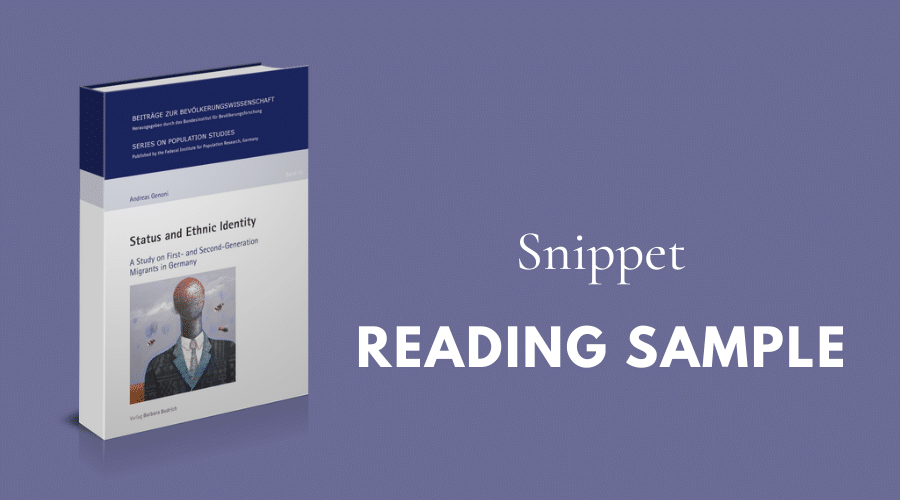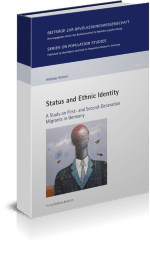# Snippet: Reading sample from “Status and Ethnic Identity“### Status and Ethnic Identity. A Study on First- and Second-Generation Migrants in Germany

by Andreas Genoni

Migrants’ minority and majority identity are controversial political topics, which disclose challenges of integration and social cohesion. Based on a two-dimensional model of ethnic identity, the book asks about the role of social status for migrants’ identification with their origin group and the majority population. It focuses on intergenerational differences, migrant visibility, status mismatch, and exposure in the receiving country. Results reveal forms of ethnic identity beyond the classical assumption of mutual exclusiveness, which suggests that minority identity primarily relates to lower status positions and majority identity to higher status positions.The book thus contributes to the important discussion about migrant assimilation and alternative forms of incorporation.

### 4 Empirical Investigation

The aim of the empirical investigation is to gain a better understanding of the relationship between migrants’ status and their ethnic identity from a bidimensional and intergenerational perspective. This is done in three distinct analyses, which are distributed over three empirical sections. Previous to the empirical sections, Section 4.1 briefly explains the use of multi-nomial logistic regression analyses, the regression technique used throughout the empirical sections to address this book’s research questions. In Section 4.1, it is also elaborated on the caveats associated with non-linear regression techniques like multinomial logistic regressions and how they are dealt with.

The empirical investigation begins with Section 4.2 and a straightforward analysis of how status is linked to ethnic identity in general. This analysis represents the point of departure for the subsequent analyses because it takes a bidimensional perspective on ethnic identity and explores its relation to status from an intergenerational point of view. The subsequent analyses then move on and investigate specific conditions that might influence the link between status and ethnic identity in the first and second generation. In this regard, an additional analysis in Section 4.2 investigates the role of migrant recognisability for how status relates to ethnic identity. One goal of this analysis is to explain the so-called “integration paradox,” which posits that particularly higher-status migrants should refrain from majority identification due to feelings of relative deprivation.

The role of status-related feelings of relative deprivation for ethnic identity is further investigated in Section 4.3. Improving the understanding of how status relates to ethnic identity also requires awareness of status discrepancies and their potential consequences on migrants’ ethnic identity. The featured analysis thus studies the ethnic identity of migrants with status mismatch. They are defined as migrants whose educational qualification exceeds the educational qualification required by their current occupation. This analysis further aims at providing another explanation to the integration paradox as it investigates whether status mismatch and ethnic identity are particularly related among higher educated migrants.

Lastly, the goal of the analysis in Section 4.4 is to investigate the relationship between
status and ethnic identity within a longer-term context by focussing on the role of migrants’ exposure time in the receiving society. The analysis therefore addresses the importance of status differences in ethnic identity in view of temporal changes of ethnic identity. All empirical sections follow the same structure: First, the theoretical arguments are outlined and an overview of the hypotheses is provided. Second, the analytical strategy for testing the hypotheses is suggested and the empirical model is specified. Third, empirical results are reported before the findings are discussed in the last step.

#### 4.1 A note on multinomial logistic regression analysis

I analyse migrants‘ ethnic identity by employing multinomial logistic regressions (Kühnel/Krebs 2010; Long 2015). In ethnic identity research, the majority of scholars view minority identity and majority identity as two independent dimensions. In this respect, migrants’ ethnic identification does not simply follow a unilinear or unidirectional path and cannot be ranked in a specific order. The four ethnic identity types that are captured by my dependent variable “ethnic identity” thus reflect a nominal scale that is best captured by the multinomial logistic regression method.

In the following analyses, I refrain from reporting odds ratios or logarithmic odds (log-odds) and instead report predicted probabilities, test statistics, and goodness of fit statistics to ease interpretation of results. Log-odds and odds ratios are the ordinary coefficients reported in non-linear regression models, as these models apply likelihood estimation techniques. However, log-odds and odds ratios are difficult to interpret (see Best/Wolf 2010; Breen et al. 2018; Mood 2010 for a discussion). They reflect coefficients which are non-linearly related to the regression outcome. It is often difficult to grasp the meaning of odds, which can cause misunderstandings and false interpretations. Moreover, log-odds and odds ratios are also biased by correlated and uncorrelated unobserved heterogeneity. Uncorrelated unobserved heterogeneity describes the phenomenon that independent variables are biased by covariates that are (a) uncorrelated with explaining variables and (b) not considered in non-linear regression models. Non-linear regressions differ from linear regressions in this regard. Independent variables in linear regressions are “only” affected by correlated unobserved heterogeneity, which refers to unobserved variables that are correlated with independent model variables. Thus, in non-linear regression models, adding previously omitted variables potentially changes the extent of overall unobserved heterogeneity and with it the log-odds and odds ratios of independent variables already in the model. As a consequence, assessing whether the added variables are correlated or uncorrelated with their independent variables turns out to be a difficult task, which increases the risk of erroneous interpretations and conclusions.

There are various solutions for these issues of which reporting predicted probabilities is by far the most important one. In non-linear regression models, predicted probabilities are obtained by calculating marginal effects. According to Mize (2 019: 84), “marginal effects summarise an independent variable’s effect in terms of a model’s predictions.” Marginal effects are more robust to interpret than odds because they are expressed and interpreted in a different metric and thus largely avoid the issue of uncorrelated unobserved heterogeneity of odds (Best/Wolf 2012; Breen et al. 2018; Long 1997; Long/Freese 2014). Mize points out that odds are in fact already a conversion of predicted probabilities. Consequently, he argues that non-linear regression results should be reported in predicted probabilities as they represent the original or “natural” metric of dependent variables.

Marginal effects are complemented by reporting average marginal effects (AMEs), which represent a specific type of marginal effects. AMEs “are estimated by calculating marginal effects for each observation in the sample and then averaging these effects” (Mize 2019: 86). As such, they refer to the additive average effects of an independent variable on the probability of a dependent variable (Best/Wolf 2012: 383). With respect to ethnic identity, AMEs would indicate average differences in ethnic identity probabilities between selected values of covariates. For example, a negative AME for education-occupation mismatch on assimilated identity for migrants would indicate that status-mismatched migrants are on average less likely to show assimilated identity compared to their non-mismatched counterparts. In contrast, a positive AME would indicate an increased probability of status-mismatched migrants to show assimilated identity compared to their non-mismatched counterparts (i.e. the reference category). Thus, while marginal effects would depict the probability to show assimilated identity for migrants with and without education-occupation mismatch, AMEs depict the difference between the marginal effects for assimilated identity between migrants with and without education-occupation mismatch.

Importantly, marginal effects and AMEs not only allow robust interpretation of the effect of binary variables in non-linear regression models. They also allow robust interpretation of the effect of continuous variables when calculated across different values of a specific independent variable. Predicted probabilities can therefore capture non-linear relationships in non-linear models. To easily assess the nature of relationships between continuous independent and dependent variables in non-linear regression models, Long (2009) and others (e.g. Best/Wolf 2010; Mize 2019) recommend graphical plotting of predicted probabilities.

In addition to predicted probabilities, there are other statistical values that ease interpretation of multinomial logistic regression results. With respect to test statistics, z-statistics allow assessing the relative effect strength of regression coefficients in non-linear regression models. By comparing z-statistics in a non-linear regression model, one can for example determine the importance of education-occupation mismatch for migrants to show separated identity compared to other variables such as migrants’ age, their cultural distance or education. In contrast to z-statistics, the Likelihood-Ratio (LR) test allows for analysing the significance of overall effects of independent variables on dependent variables. This is done by comparing so-called nested models. Nested models refer to models of which one is basically an extension of the other as it includes additional variables. Goodness of fit statistics such as pseudo-R2 measures, the Akaike Information Criterion (AIC) or Bayesian Information Criterion (BIC) indicate how strong a specific independent variable affects the dependent variable by comparing models with and without the respective independent variable(s). These statistics thus provide information on the importance of specific independent variables for explaining a dependent variable. Importantly, AIC and BIC also enable comparisons between un-nested models. They thus allow the comparison of relationships between independent and dependent variables across migrant generations, even though generation-specific regression models are estimated.

Considering the outlined statistical repertoire that is available to researchers, I will report various statistical indicators including test statistics, goodness of fit statistics and predicted probabilities to ensure valid interpretation of the results in this book. Furthermore, I will investigate outcomes on ethnic identity graphically by plotting its predicted probabilities in profile plots and conditional effect plots (see Bauer 2010). Overall, these solutions enable me to test various hypotheses by investigating the direction that variables affect each other, relative effect strengths and overall effects of my explaining variables as well as non-linear and interaction effects. Moreover, I am able to compare coefficients across models and groups, which is crucial for analysing structural and intergenerational differences in migrants’ ethnic
identity.

***

Would you like to read more?

###Order via our webshop

Status and Ethnic Identity. A Study on First- and Second-Generation Migrants in Germany

by Andreas Genoni